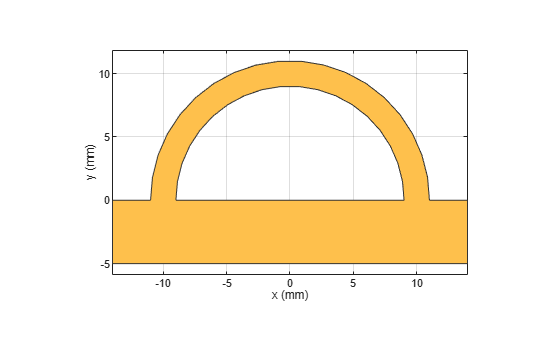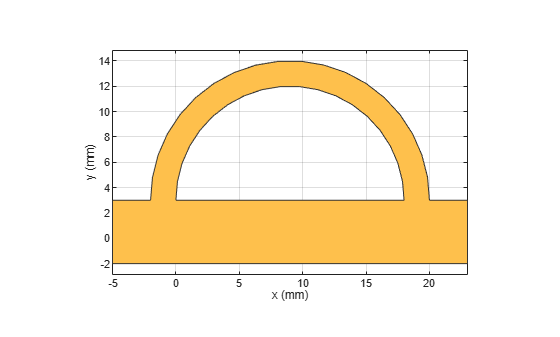# translate

Move RF PCB shape to new location

Since R2021b

## Syntax

``c = translate(shape,offset)``

## Description

example

````c = translate(shape,offset)` moves the shape to a new specified location using a translation vector.```

## Examples

collapse all

Create a shape consisting of a curve and a right angle U-bend.

```shape1 = curve; shape2 = ubendRightAngle(Length=[5 18 5]*1e-3,ReferencePoint=[0 -5]*1e-3); shapeSum = shape1+shape2;```

Display the shape.

`show(shapeSum)`Specify a translation vector, then translate the shape in the X-Y plane. Display the result.

```t = [9 3 0]*1e-3; shapeTrans = translate(shapeSum,t); show(shapeTrans)```## Input Arguments

collapse all

RF PCB shape created using custom elements and shape objects of RF PCB Toolbox™, specified as an object.

Example: `shape = bendCurved;` specifies the shape as a `bendCurved` object.

Translation vector, specified as a vector.

Data Types: `double`

## Version History

Introduced in R2021b